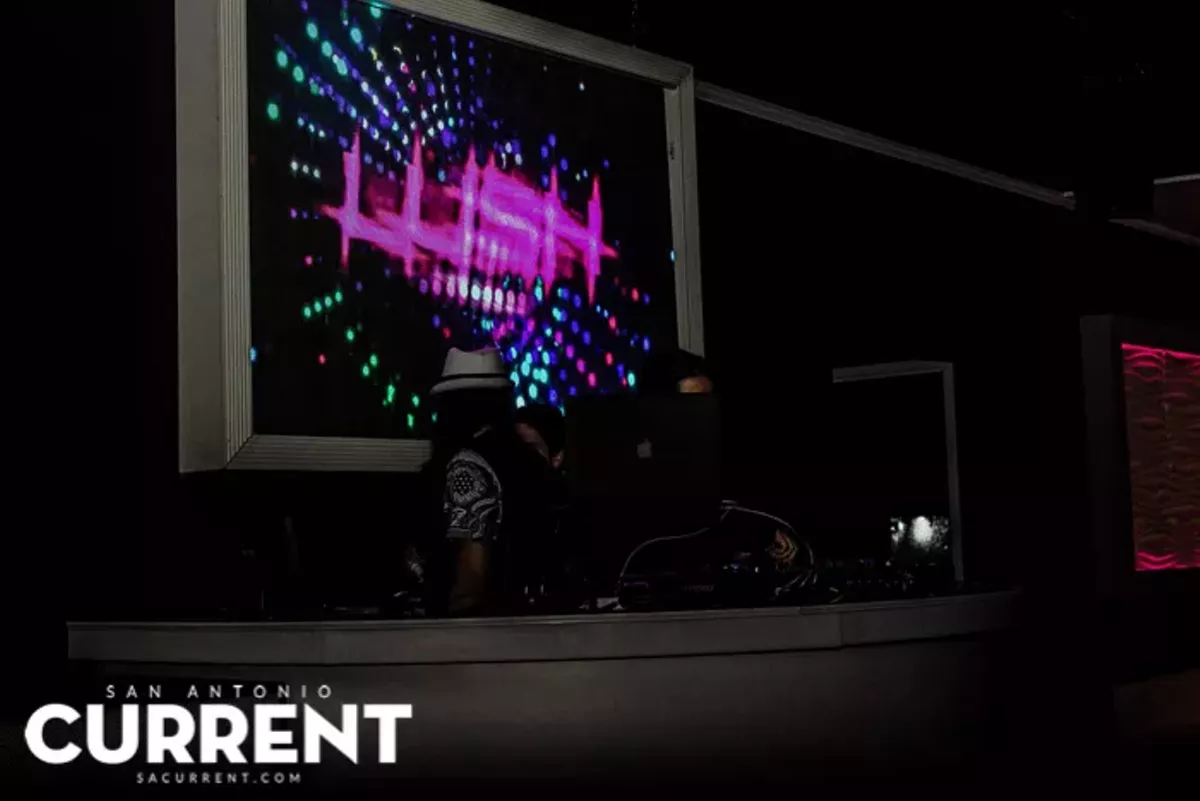# 25 Shots of Lush’s Grand Opening Party

Tweet
Submit to Reddit
Email
11/13/2015
Photos by B. K-McGee
OF 32
PREV NEXTB. K-McGee
B. K-McGee
B. K-McGee
B. K-McGee
B. K-McGee
B. K-McGee
B. K-McGee
B. K-McGee
B. K-McGee
B. K-McGee
B. K-McGee
B. K-McGee
B. K-McGee
B. K-McGee
B. K-McGee
B. K-McGee
B. K-McGee
B. K-McGee
B. K-McGee
B. K-McGee
B. K-McGee
B. K-McGee
B. K-McGee
B. K-McGee
B. K-McGee
B. K-McGee
B. K-McGee
B. K-McGee
B. K-McGee
B. K-McGee